# 程序员最近都爱上了这个网站  程序员们快来瞅瞅吧！  it98k网:it98k.com出租广告位,需要合作请联系站长

+关注

2021-10(5)

2021-11(16)

## Pandas向本地Excel已存在的工作表追加写入DataFrame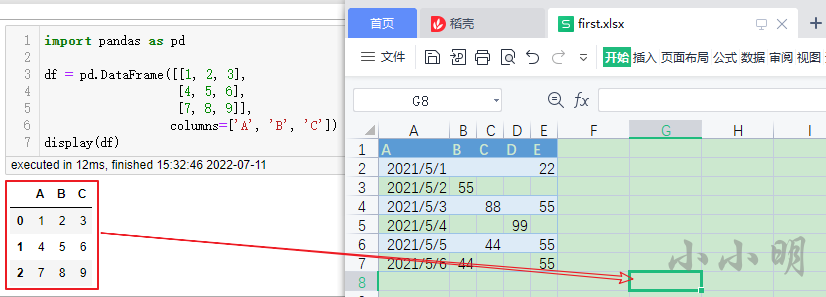`Once a workbook has been saved it is not possible write further data without rewriting the whole workbook.`

``````import pandas as pd

df = pd.DataFrame([[1, 2, 3],
[4, 5, 6],
[7, 8, 9]],
columns=['A', 'B', 'C'])
``````

## openpyxl向工作表追加Pandas数据

``````from openpyxl import load_workbook

sheet = book.active

for j, v in enumerate(df.columns, 1):
sheet.cell(start_row+1, start_col+j).value = v
for i, row in enumerate(df.values, 2):
for j, v in enumerate(row, 1):
sheet.cell(start_row+i, start_col+j).value = v
book.save("test.xlsx")
``````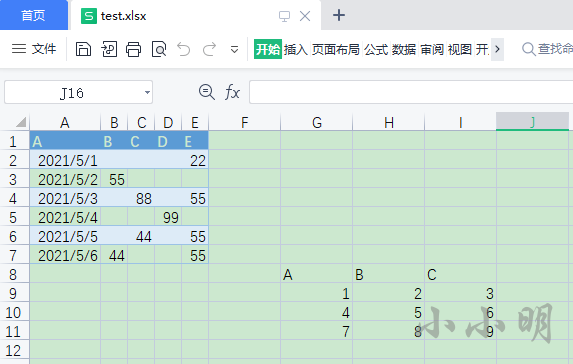## xlwings向工作表追加Pandas数据

``````import xlwings as xw

wb = app.books.open("first.xlsx")
sht = wb.sheets.active
sht.range('G8').options(index=False).value = df
wb.save("test.xlsx")
app.quit()
``````

options参数可以设置DataFrame的写出形式，与to_excel的参数几乎一致。

## Pandas直接向工作表追加数据

``````writer = pd.ExcelWriter("first.xlsx", engine='openpyxl', mode='a')
writer.sheets.update({sht.title: sht for sht in writer.book.worksheets})
df.to_excel(writer, sheet_name=writer.book.active.title,
index=False, startrow=7, startcol=6)
writer.close()
``````

``````writer = pd.ExcelWriter("first.xlsx", engine='openpyxl',
mode='a', if_sheet_exists="overlay")
df.to_excel(writer, sheet_name=writer.book.active.title,
index=False, startrow=7, startcol=6)
writer.close()
``````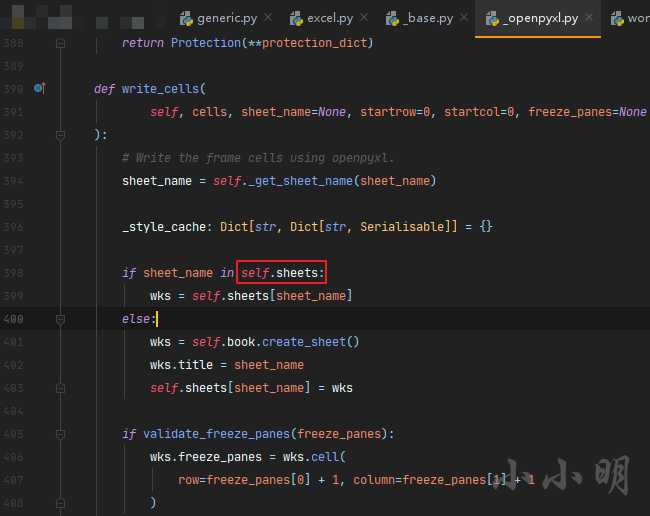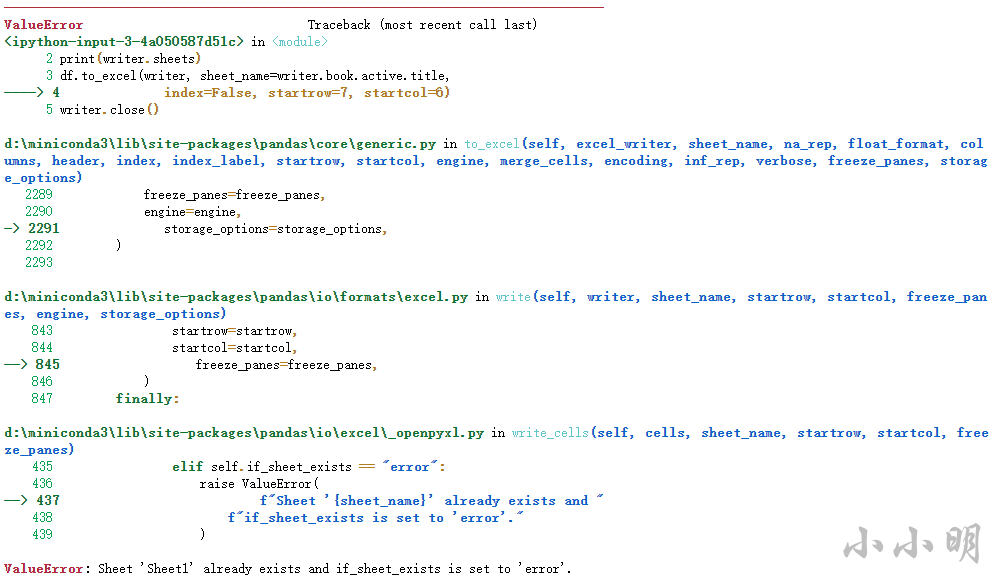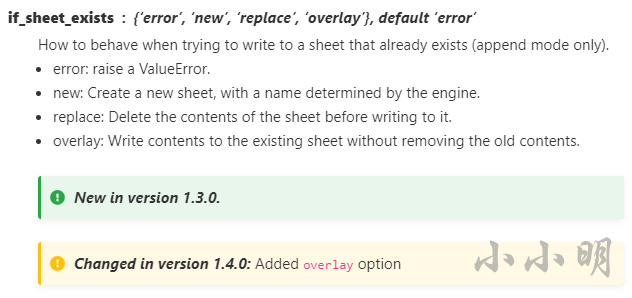``````writer = pd.ExcelWriter("first.xlsx", engine='openpyxl',
mode='a', if_sheet_exists="overlay")
df.to_excel(writer, sheet_name=writer.book.active.title,
index=False, startrow=7, startcol=6)
writer.close()
``````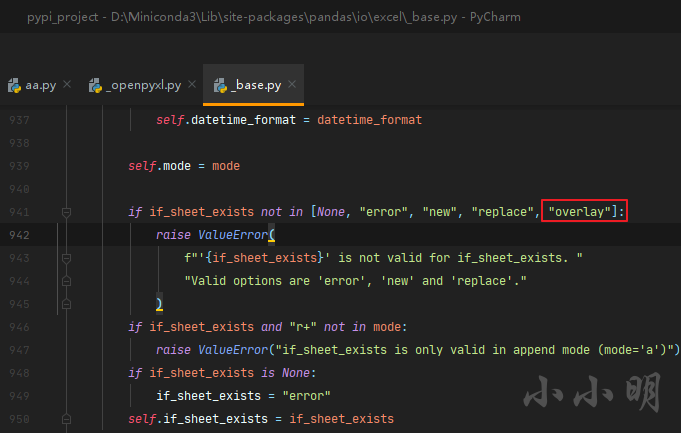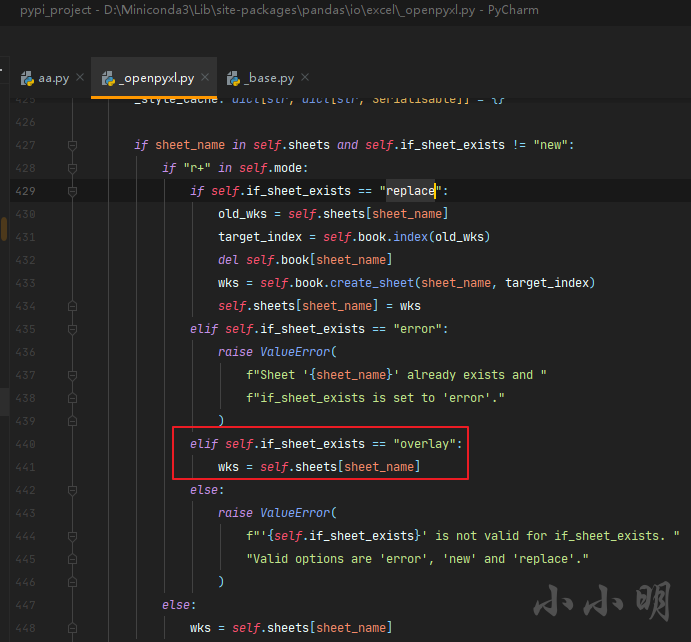3 0

pdf(new) 更多>